Test: Direct Current Machine of Electrical Machines

# Test: Direct Current Machine of Electrical Machines

Test Description

## 10 Questions MCQ Test GATE Electrical Engineering (EE) 2023 Mock Test Series | Test: Direct Current Machine of Electrical Machines

Test: Direct Current Machine of Electrical Machines for Electrical Engineering (EE) 2023 is part of GATE Electrical Engineering (EE) 2023 Mock Test Series preparation. The Test: Direct Current Machine of Electrical Machines questions and answers have been prepared according to the Electrical Engineering (EE) exam syllabus.The Test: Direct Current Machine of Electrical Machines MCQs are made for Electrical Engineering (EE) 2023 Exam. Find important definitions, questions, notes, meanings, examples, exercises, MCQs and online tests for Test: Direct Current Machine of Electrical Machines below.
Solutions of Test: Direct Current Machine of Electrical Machines questions in English are available as part of our GATE Electrical Engineering (EE) 2023 Mock Test Series for Electrical Engineering (EE) & Test: Direct Current Machine of Electrical Machines solutions in Hindi for GATE Electrical Engineering (EE) 2023 Mock Test Series course. Download more important topics, notes, lectures and mock test series for Electrical Engineering (EE) Exam by signing up for free. Attempt Test: Direct Current Machine of Electrical Machines | 10 questions in 30 minutes | Mock test for Electrical Engineering (EE) preparation | Free important questions MCQ to study GATE Electrical Engineering (EE) 2023 Mock Test Series for Electrical Engineering (EE) Exam | Download free PDF with solutions
 1 Crore+ students have signed up on EduRev. Have you?
Test: Direct Current Machine of Electrical Machines - Question 1

### Consider the following statements about the field windings used for various types of dc machines: 1. For dc shunt machines, large number of turns of small cross-section are used. 2. For dc series machine, small number of turns of large cross-section are used. 3. For dc compound machine, both shunt (thick wire) and series (thin wire) field windings are used. Out of the above statements the correct statements is/are:

Detailed Solution for Test: Direct Current Machine of Electrical Machines - Question 1

For dc shunt machine, field current is very less, therefore for required field AT/Pole, number of turns of field winding is increased. Thus, R should be high for low If.
For dc series machine field winding current is equal to armature current which is of larger value. Therefore, number of turns of field winding is reduced to get the required field AT/Pole. As it has to handle large current, therefore resistance of field winding should be less by keeping the winding thick. Statement-3 is wrong.

Test: Direct Current Machine of Electrical Machines - Question 2

### A 6-pole dc machine has 300 conductors and flux per pole is 15 mWb, If the machine Is driven at 1800 rpm, then the average emf induced per conductor will be

Detailed Solution for Test: Direct Current Machine of Electrical Machines - Question 2

Given, speed,
N= 1800 rpm
n = 1800/60 rps = 30 rps
Average emf induced per conductor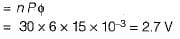Test: Direct Current Machine of Electrical Machines - Question 3

### A commutator with a diameter of 50 cm rotates at 1000 rpm. For a brush width of 1.5 cm, the time of commutation is

Detailed Solution for Test: Direct Current Machine of Electrical Machines - Question 3

Peripheral velocity of commutator is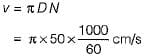Now, brush width = v x time of commutation

or, time of commutation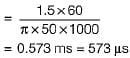Test: Direct Current Machine of Electrical Machines - Question 4

A 24 kW, 250 V, 1600 rpm separately-excited d.c. generator has armature circuit resistance of 0.1Ω. The machine is first run at rated speed and the field winding current is adjusted to give an open circuit voltage of 260 V. Now, when the generator is loaded to deliver its rated current, the speed of the driving motor is found to be 1500 r.p.m. Assuming flux to remain constant, the terminal voltage of the generator under these conditions is

Detailed Solution for Test: Direct Current Machine of Electrical Machines - Question 4

We know that,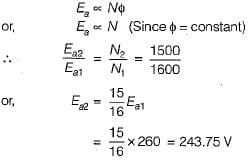Now, rated armature current is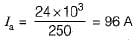So, the terminal voltage is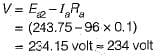Test: Direct Current Machine of Electrical Machines - Question 5

Assertion (A): DC series motors are suitable for buses, trolleys, hoists and trains.
Reason (R): DC series motors are variable Speed motors.

Detailed Solution for Test: Direct Current Machine of Electrical Machines - Question 5

The reason of assertion is that dc series motor develop high torque at start due to which these motors are used for hard to start loads. Reason is also correct because these are variable speed motors.

Test: Direct Current Machine of Electrical Machines - Question 6

Assertion (A): Armature resistance control method of speed control of dc motors is wasteful and inefficient.
Reason (R): Large amount of power is wasted in the control resistances, specially in case of shunt motors'.

Test: Direct Current Machine of Electrical Machines - Question 7

Match List-I (Tests on DC machines) with
List-ll (Results) and select the correct answer using the codes given below the lists:
List-I
A. Field test
B. Swinburne’s test
C. Hopkinson test
D. Retardation test
List-II
1. Stray losses in case of dc series machines
2. Constant losses
3. Performance of machines regarding commutation and temperature rise
4. Stray losses in case of dc shunt machines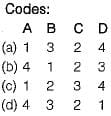Test: Direct Current Machine of Electrical Machines - Question 8

At a certain speed and flux, the voltage generated by a dc generator is 230 volts. If the speed is increased by 20% and the flux is simultaneously reduced by 10%, the voltage generated will be

Detailed Solution for Test: Direct Current Machine of Electrical Machines - Question 8

We know that,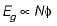∴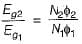or,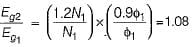∴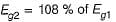Thus, voltage generated is increased by 8%.

Test: Direct Current Machine of Electrical Machines - Question 9

LAP winding is employed in a dc machine of

Detailed Solution for Test: Direct Current Machine of Electrical Machines - Question 9

For LAP winding, number of parallel path = number of poles.
Thus, resistance is less compared to wave winding. Hence, current rating is more and voltage rating is less compared to wave winding.

Test: Direct Current Machine of Electrical Machines - Question 10

Consider the following parts of a dc machine:
1. Pole core
2. Brushes
3. Yoke
4. Armature core
Which of the above parts are not subjected to iron loss?

## GATE Electrical Engineering (EE) 2023 Mock Test Series

22 docs|274 tests
Information about Test: Direct Current Machine of Electrical Machines Page
In this test you can find the Exam questions for Test: Direct Current Machine of Electrical Machines solved & explained in the simplest way possible. Besides giving Questions and answers for Test: Direct Current Machine of Electrical Machines, EduRev gives you an ample number of Online tests for practice

## GATE Electrical Engineering (EE) 2023 Mock Test Series

22 docs|274 tests(Scan QR code)